# [CVPR2020/PaperSummary]Point-GNN: Graph Neural Network for 3D Object Detection in a Point Cloud

1. Introduction
1. Graph construction
2. GNN of T iterations
3. Bounding box merging and scoring
• graph radius with r = 1.6m.
• Pˆ as a downsampled point cloud by a voxel size of 0.4 meters in training and 0.2 meters in inference.
• M LPf and M LPg, are both of sizes (256, 256).
• For the initial vertex state, M LP is of (32, 64, 128,256,512) for embedding raw points and another M LP of (256, 256) after the M ax aggregation.
• NMS threshold = 0.2 in NMS
• Batchsize = 4
• The loss weights are α = 0.1, β = 10, γ = 5e — 7.
• stochastic gradient descent (SGD) with a stair-case learning-rate decay.
• For Car, we use an initial learning rate of 0.125 and a decay rate of 0.1 every 400K steps.
• For Pedestrian and Cyclist, we use an learning rate of 0.32 and a decay rate of 0.25 every 400K steps.
• Global rotation, global flipping, box translation and vertex jitter.
• During training, random rotation of the point cloud by yaw ∆θ ∼ N (0, π/8) and then flip the x-axis by a probability of 0.5. After that, each box and points within 110% size of the box randomly shift by (∆x ∼ N (0, 3), ∆y = 0, ∆z ∼ N (0, 3)).
• During the translation, we check and avoid collisions among boxes, or between background points and boxes.
• During graph construction, we use a random voxel downsample to induce vertex jitter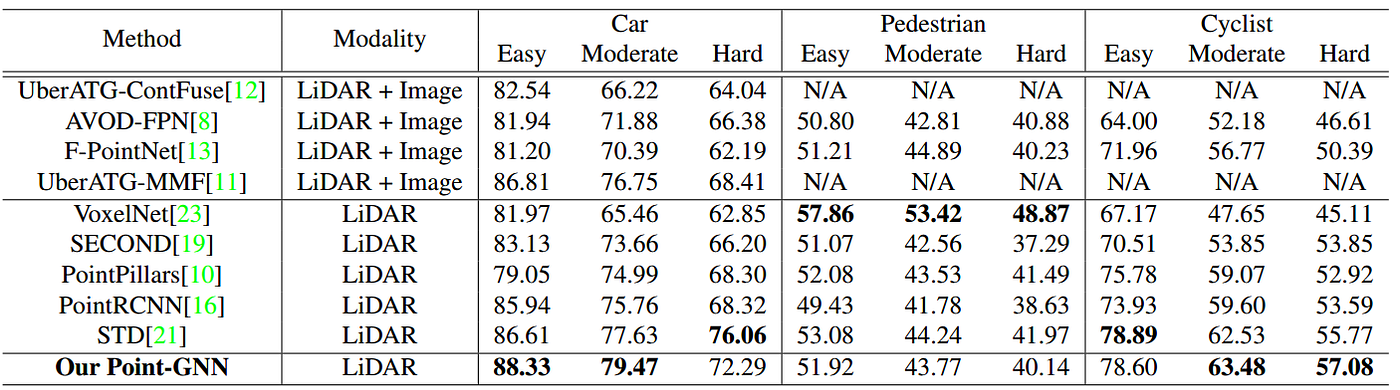Table 1. The Average Precision (AP) comparison of 3D object detection on the KITTI test dataset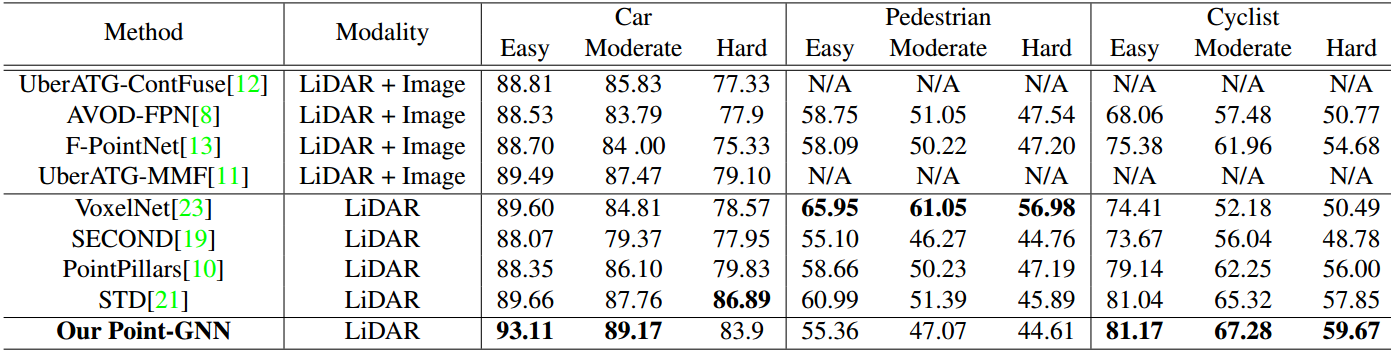Table 2. The Average Precision (AP) comparison of Bird’s Eye View (BEV) object detection on the KITTI test dataset.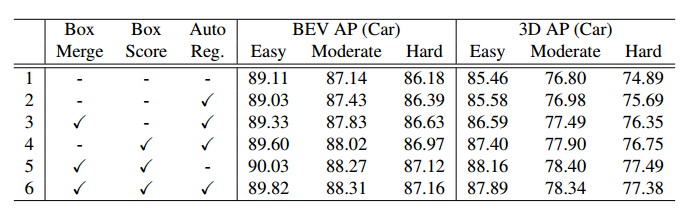Table 3. Ablation study on the val. split of KITTI data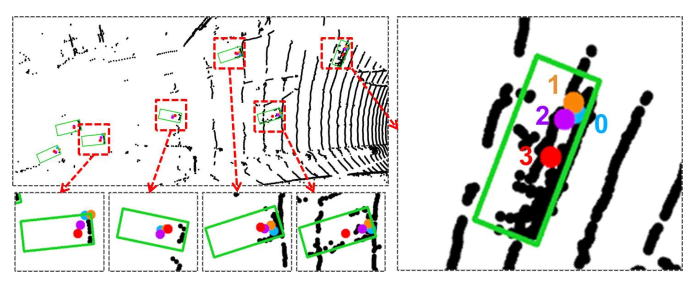Figure3 : The blue dot indicates the original position of the vertices. The orange, purple, and red dots indicate the original position with added offsets from the first, the second, and the third graph neural network iterations. Best viewed in color.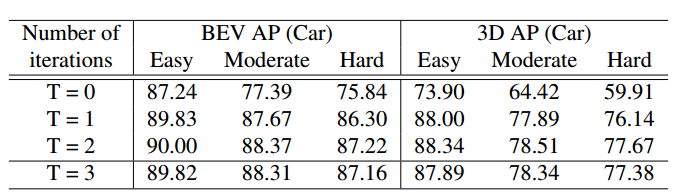Table 4. Average precision on the KITTI val. split using differentnumber of Point-GNN iterations.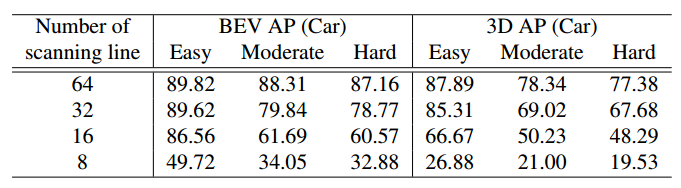Table 5. Average precision on downsampled KITTI val. split
• A novel work of 3D object detection using GNN
• Auto registration and refinement module implemented
• Accuracy drops considerably for Large Range and sparse point cloud .
• High latency time ie low fps.

--

--